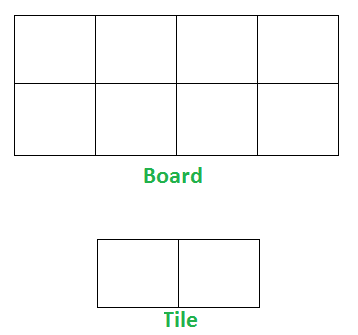GFG App
Open AppBrowser
Continue

# Tiling Problem

Given a “2 x n” board and tiles of size “2 x 1”, count the number of ways to tile the given board using the 2 x 1 tiles. A tile can either be placed horizontally i.e., as a 1 x 2 tile or vertically i.e., as 2 x 1 tile.

Examples:

Input: n = 4
Output: 5
Explanation:
For a 2 x 4 board, there are 5 ways

• All 4 vertical (1 way)
• All 4 horizontal (1 way)
• 2 vertical and 2 horizontal (3 ways)

Input: n = 3
Output: 3
Explanation:
We need 3 tiles to tile the board of size  2 x 3.
We can tile the board using following ways

• Place all 3 tiles vertically.
• Place 1 tile vertically and remaining 2 tiles horizontally (2 ways)Implementation –

Let “count(n)” be the count of ways to place tiles on a “2 x n” grid, we have following two ways to place first tile.
1) If we place first tile vertically, the problem reduces to “count(n-1)”
2) If we place first tile horizontally, we have to place second tile also horizontally. So the problem reduces to “count(n-2)”
Therefore, count(n) can be written as below.

```   count(n) = n if n = 1 or n = 2
count(n) = count(n-1) + count(n-2)```

Here’s the code for the above approach:

## C++

 `// C++ program to count the` `// no. of ways to place 2*1 size` `// tiles in 2*n size board.` `#include ` `using` `namespace` `std;`   `int` `getNoOfWays(``int` `n)` `{` `    ``// Base case` `    ``if` `(n <= 2)` `      ``return` `n;`   `    ``return` `getNoOfWays(n - 1) + getNoOfWays(n - 2);` `}`   `// Driver Function` `int` `main()` `{` `    ``cout << getNoOfWays(4) << endl;` `    ``cout << getNoOfWays(3);` `    ``return` `0;` `}`

## Java

 `/* Java program to count the` ` ``no of ways to place 2*1 size` ` ``tiles in 2*n size board. */` `import` `java.io.*;`   `class` `GFG {` `  ``static` `int` `getNoOfWays(``int` `n)` `  ``{`   `    ``// Base case` `    ``if` `(n <= ``2``) {` `      ``return` `n;` `    ``}` `    ``return` `getNoOfWays(n - ``1``) + getNoOfWays(n - ``2``);` `  ``}`   `  ``// Driver Function` `  ``public` `static` `void` `main(String[] args)` `  ``{` `    ``System.out.println(getNoOfWays(``4``));` `    ``System.out.println(getNoOfWays(``3``));` `  ``}` `}`   `// This code is contributed by ashwinaditya21.`

## Python3

 `# Python3 program to count the` `# no. of ways to place 2*1 size` `# tiles in 2*n size board.` `def` `getNoOfWays(n):` `  `  `    ``# Base case` `    ``if` `n <``=` `2``:` `        ``return` `n`   `    ``return` `getNoOfWays(n ``-` `1``) ``+` `getNoOfWays(n ``-` `2``)`   `# Driver Code` `print``(getNoOfWays(``4``))` `print``(getNoOfWays(``3``))`   `# This code is contributed by Kevin Joshi`

## C#

 `// C# program to implement` `// the above approach` `using` `System;`   `class` `GFG` `{` `    `  `static` `int` `getNoOfWays(``int` `n)` `  ``{` ` `  `    ``// Base case` `    ``if` `(n <= 2) {` `      ``return` `n;` `    ``}` `    ``return` `getNoOfWays(n - 1) + getNoOfWays(n - 2);` `  ``}`   `// Driver Code` `public` `static` `void` `Main()` `{` `    ``Console.WriteLine(getNoOfWays(4));` `    ``Console.WriteLine(getNoOfWays(3));` `}` `}`   `// This code is contributed by code_hunt.`

## Javascript

 ``

Output

```5
3```

Time Complexity: O(2^n)
Auxiliary Space: O(1)

The above recurrence is nothing but Fibonacci Number expression. We can find n’th Fibonacci number in O(Log n) time, see below for all method to find n’th Fibonacci Number.
https://youtu.be/NyICqRtePVs
https://youtu.be/U9ylW7NsHlI
Different methods for n’th Fibonacci Number
Count the number of ways to tile the floor of size n x m using 1 x m size tiles